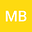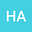MHD hybrid-nanofluid (CNT - Fe3O4-H2O) flow through a squeezing/dilating walls of the asymmetric channel
••The fundamental concern of the present study is to analyze the magnetohydrodynamics (MHD) hybrid nanofluid (Carbon-nanotubes and ferrous oxide-water)$CNT-Fe_{3}O_{4}/H_{2}O$ flow through a horizontal parallel channel having squeezing and dilating porous walls along with thermal radiation. The porous walls of the channel cause the parting motion. The fluid flow is laminar and time-dependent. The channel is asymmetric and the temperature and porosity of the upper and lower walls are different. The concept of hybrid nanofluid is exploited with the combination of nanoparticles of $Fe_{3}O_{4}$ and single and multi-wall carbon nanotubes. The set of partial differential equations (PDEs) of this mathematical model, governed from momentum and energy equations, are reduced to respective ordinary differential equations (ODEs) by using the similarity transformation. To achieve the solutions of governing ODEs, a very common numerical approach called, Runge-Kutta method of order four along with shooting technique is utilized. A computing software MATLAB is used to construct the graphs of temperature and velocity profiles for different emerging parameters. The main findings are summarized at the end of the manuscript.